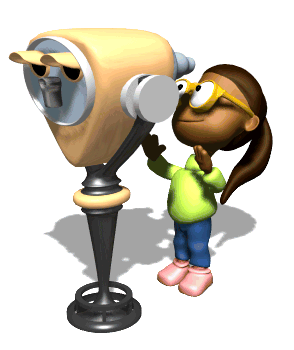# Optics

Light is an electromagnetic wave, and can reflect, refract, and diffract. By understanding how light (and other waves) behave at interfaces and boundaries, we can not only understand a wide variety of natural phenomena, but also harness these waves for use in a variety of applications, ranging from eye glasses, binoculars and telescopes to manufacturing, medicine, and microelectronics!

### Objectives

1. Explain the law of reflection.
2. Analyze the behavior of plane and curved mirrors.
3. Understand and apply Snell’s law.
4. Calculate the index of refraction in a medium.
5. Determine the critical angle for an interface.
6. Analyze the behavior of convex and concave lenses.
7. Relate the diffraction of light to its wave characteristics.
8. Describe Young’s double-slit experiment.
9. Recognize characteristics of EM waves and determine the type of EM wave based on its characteristics.